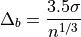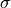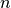# 11.2.1.4. astroML.density_estimation.scotts_bin_width¶

astroML.density_estimation.scotts_bin_width(data, return_bins=False)[source]

Deprecated since version 0.4: The scotts_bin_width function is deprecated and may be removed in a future version. Use astropy.stats.scott_bin_width instead.

Return the optimal histogram bin width using Scott’s rule:

Parameters
dataarray-like, ndim=1

observed (one-dimensional) data

return_binsbool (optional)

if True, then return the bin edges

Returns
widthfloat

optimal bin width using Scott’s rule

binsndarray

bin edges: returned if return_bins is True

Notes

The optimal bin width iswhereis the standard deviation of the data, andis the number of data points.